Function Repository Resource:

# SymbolicSort

Sort symbolic expressions using assumptions

Contributed by: Sjoerd Smit
 ResourceFunction["SymbolicSort"][list,vars] attempts to sort list by assuming that the variables vars are real-valued. ResourceFunction["SymbolicSort"][list,vars,assum] sorts by assuming that vars satisfy assumptions assum. ResourceFunction["SymbolicSort"][list,vars,assum,dom] carries out symbolic manipulations over the domain dom. ResourceFunction["SymbolicSort"][list,vars,assum,dom,List] always returns a List, even if some elements do not have a definite ordering. ResourceFunction["SymbolicSort"][list,vars,assum,dom,Graph] returns a Graph showing the order relations found between the elements of list, including elements that do not have a definite ordering.

## Details and Options

The argument vars can be either a List or a single variable.
ResourceFunction["SymbolicSort"] uses Resolve and ForAll to determine if one expression is uniformly greater than another.
The default value for assum is \$Assumptions.
The default domain is Reals.
If no definite ordering can be found, ResourceFunction["SymbolicSort"] remains unevaluated and raises a message. Use ResourceFunction["SymbolicSort"][list,vars,assum,dom,List] to force the function to return a List.
In the Graph produced by ResourceFunction["SymbolicSort"][,Graph], undirected edges will be used to indicate that two expressions cannot be ordered. Directed edges will only be drawn between consecutively ordered elements. To calculate the Graph, all possible comparisons between all elements will be made, so this functionality can be slow for large lists.
A TimeConstraint (default 5) option value can be specified.

## Examples

### Basic Examples (3)

Sort expressions in x defined for real numbers:

 In:=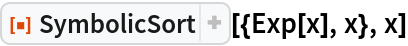Out=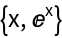Plot to illustrate that Exp[x] is always greater than x:

 In:=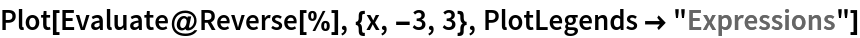Out=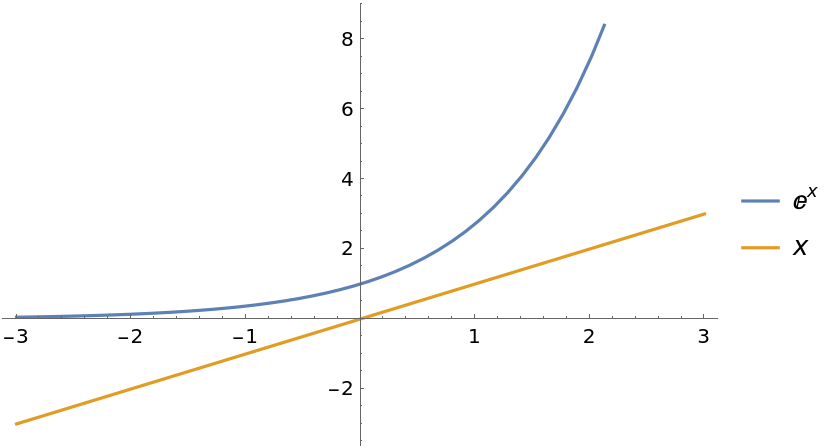Use assumptions for sorting:

 In:=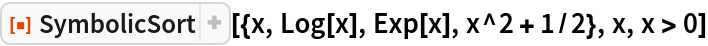Out=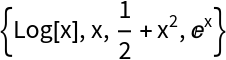In:=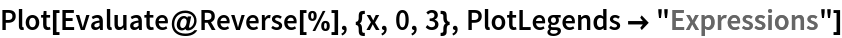Out=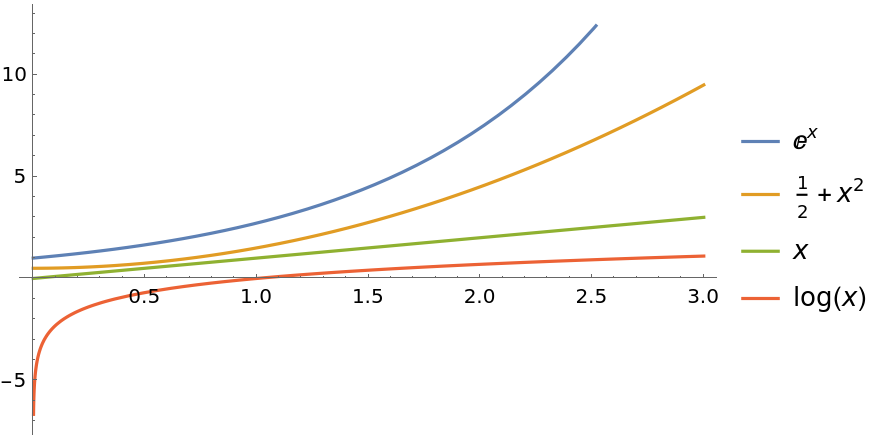Sort expressions of a monotonic function applied to different variables:

 In:=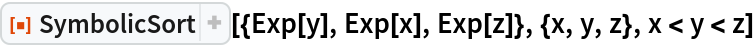Out=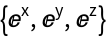### Scope (2)

If no definite order exists, a message will be raised and SymbolicSort remains unevaluated:

 In:=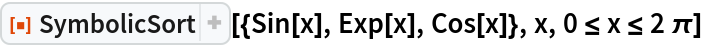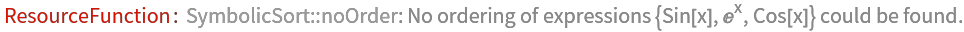Out=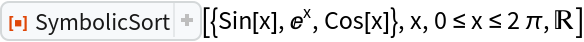Use List as the last argument to force a result:

 In:=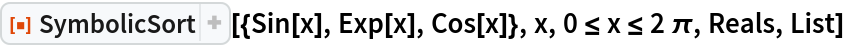Out=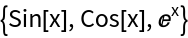If not all expressions have a definite ordering, you can instead produce a Graph that shows the ordering relationships between the elements of the List:

 In:=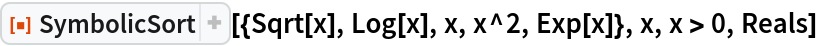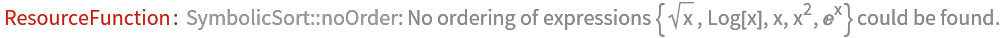Out=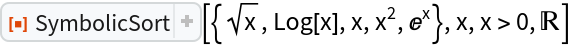In:=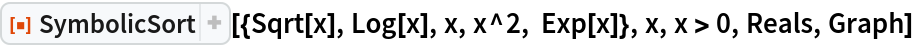Out=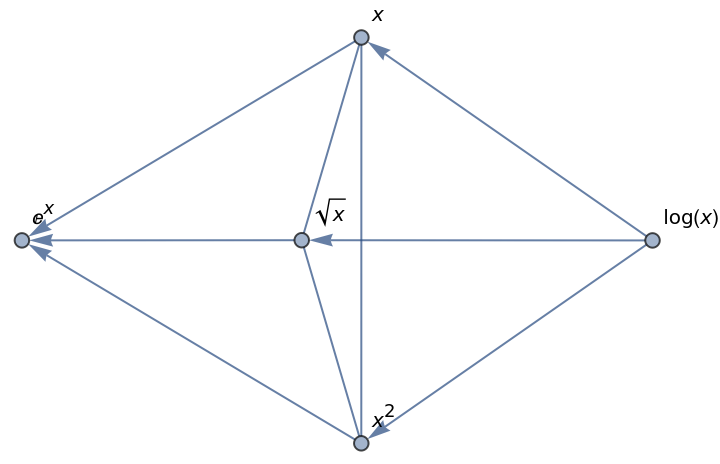The undirected edges between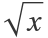, x and x2 show that these expressions have no definite ordering.

### Options (1)

#### TimeConstraint (1)

A time constraint can be set for symbolic comparisons. If the time constraint is too low, no result will be found:

 In:=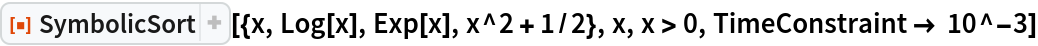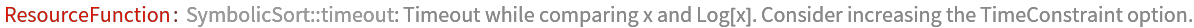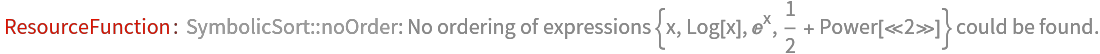Out=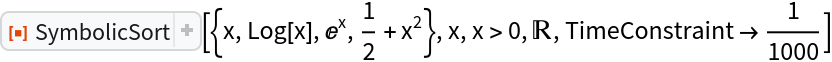### Applications (1)

Sort the eigenvalues of a symbolic matrix:

 In:=Out=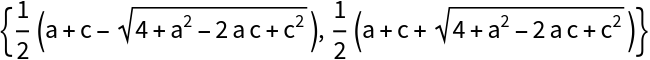Sjoerd Smit

## Version History

• 1.0.0 – 14 October 2020